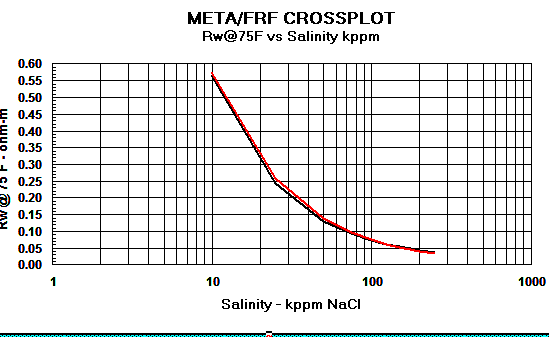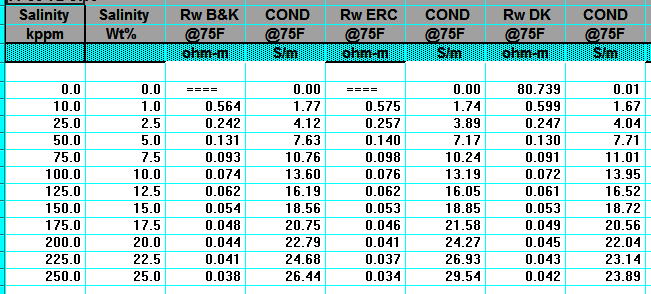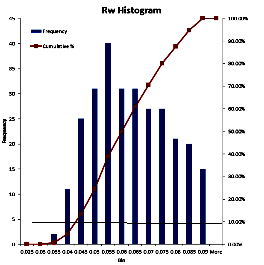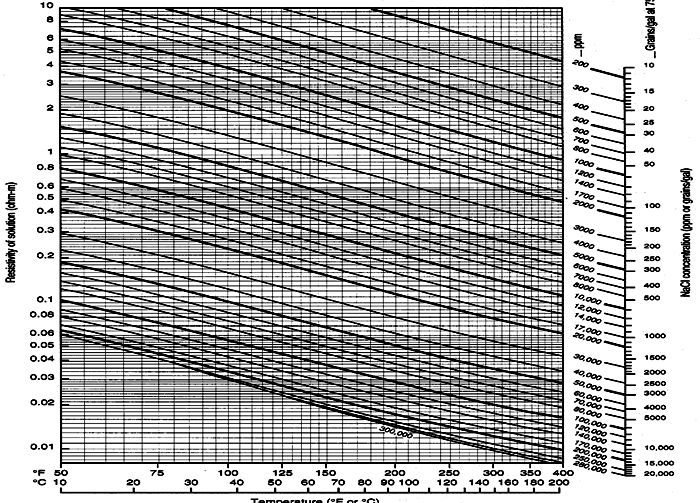Water Resistivity TRANSFORMS
Water resistivity, salinity, and temperature are related to each other with various models, described on this page. Estimating temperature is covered in the next Section of this Chapter.Water Resistivity from Salinity AT ANY TEMPERATURE
Crain's Model is used to convert a lab measured salinity to a formation water resistivity (RW) at any specific temperature (FT) in degrees Fahrenheit. The result is abbreviated as
RW@FT throughout this Handbook. You can use equation 5 to convert a salinity to any arbitrary temperature, for example 75F or 77F (roughly 25C) to find the resistivity at laboratory conditions.
1: FT = SUFT + (BHT - SUFT) / BHTDEP * DEPTH
2: IF LOGUNITS\$ = "METRIC"
3: THEN FT1 = 9 / 5 * FT + 32
4: OTHERWISE FT1 = FT
5: RW@FT = (400000 / FT1 / WS) ^ 0.88SALINITY FROM WATER RESISTIVITY
Invert Crain's equation to solve for WS given RW at a a specific temperature FT1.
6: WS = 400000 / FT1 / ((RW@ET) ^ 1.14)

Where:
BHT = bottom hole temperature (degrees Fahrenheit or Celsius)
BHTDEP = depth at which BHT was measured (feet or meters)
DEPTH = mid-point depth of reservoir (feet or meters)
FT = formation temperature (degrees Fahrenheit or Celsius)
FT1 = formation temperature (degrees Fahrenheit)
RW@FT = water resistivity at formation temperatures (ohm-m)
SUFT = surface temperature (degrees Fahrenheit or Celsius)
WS = water salinity (ppm NcCl)COMMENTS:
Use this relation if salinity is known from laboratory measurements to obtain RW from lab data at any temperature.NUMERICAL EXAMPLE:
1. Salinity to water resistivity.
RW@FT = (400000 / 102'F / 20,000 ppm) ^ 0.88 = 0.238 ohm-m @ 102'F
(rounded to three significant digits)

2. Water resistivity to salinity.
WS = 400,000 / 102'F / ((0.250 ohm-m) ^ 1.14) = 19,000 ppm NaCl
(rounded to three significant digits)Water Resistivity from Salinity at Lab Temperature
These models generate RW at laboratory temperature of 75F or 25C.
Crain's Method (1974)
1: RW@75F = (400000 / 75 / WS) ^ 0.88

Bateman and Konen Method (1977)
2: RW@75F = 0.0123 + (3647.5 / WS^0.955)

Kennedy's Method (2015)
3:  RW@75F = 1 / (24.30853 - 0.0364 * (0.1 * WS - 29.46515957) - 0.02922 * (0.1 * WS - 29.46515957)^2)SALINITY FROM WATER RESISTIVITY
Crain's Method (1974)
4: WS = 400000 / 75 / ((RW@75) ^ 1.14)

Baker Atlas Method (2002)

5: WS = 10 ^ ((3.562 - (Log (RW@75 - 0.0123))) / 0.955)COMMENTS:
For all practical purposes, the three models give the same RW value (see Graph 1 below). There are minor differences above 150,000 ppm NaCl which can only be seen when water resistivity is converted to water conductivity (see Graph 2 below).

The effect on water saturation (SW%) is not very significant (+/- 0.5% SW at low SW, +/- 2% SW at high SW, at 200,000 ppm NaCl).

The 10 significant digits used in the Kennedy equation give a false sense of accuracy that is not warranted.

SPR-08 META/LOG WATER SALINITY (WS) CALCULATOR
Calculate water salinity (WS), 3 methodsGraph 1: Rw Models - Red line = Crain, Black line = Bateman and Konen, Blue line = KennedyGraph 2: Cw Models - Red line = Crain, Black line = Bateman and Konen, Blue line = Kennedy.
The differences above 150,000 ppm NaCl have little impact on water saturation.NUMERICAL EXAMPLE:ADJUSTING RW TO FORMATION TEMPERATURE - ARP'S EQUATION
ALSO convert RW @ any temperature to any other temperature

Water resistivity (RW) data can be found in RW catalogs, drill stem test and production test reports, and water chemistry reports from a laboratory. The RW is recorded at a temperature (SUFT) that differs from the formation temperature (FT) where the log analysis is to be perforned, so an adjustment is needed. You may already have an RW value at a particular depth and temperature and want to adjust it to a different depth and temperature. The math in this Section performs these tasks..

Water catalogues published by your local well logging society or similar catalogues created by searching in-house data bases are a necessary tool for well log analysis. A sample is shown below.A sample of RW data from a water resistivity catalog, data is tabulated and also
posted on a map, and is based on a standard temperature of 25 degrees Celsius (77 degrees Fahrenheit).Water resistivity values in a catalog or a water analysis report are recorded at a standard temperature, usually 75F or 77F (25C). Since water resistivity varies inversely with temperature, the catalog values must be transformed to a new value representing water resistivity at formation temperature (RW@FT) -- see next Section.

To use data from a water catalogue, it is usually necessary to do a little filtering. Nearly everything that can go wrong will raise the RW value recorded in the catalogue. Usually the minimum value from nearby offset wells is the best choice. It may be useful to gather all the values for the reservoir for a radius of 3 to 6 miles (5 to 10 Km) and prepare a histogram. On the histogram, find the point that represents the lower decile (10% of the data values are less than this value). Take the average of the data in this decile. You may want to eliminate obvious "impossible" values before you make the histogram.

The following relationships are needed to manipulate water resistivity data prior to calculations of water saturation.

1. Arps Method (1953)
1: FT = SUFT + (BHT - SUFT) / BHTDEP * DEPTH
2: KT1 = 6.8 for English units    KT1 = 21.5 for Metric units
3: RW@FT = RW@TRW * (TRW + KT1) / (FT + KT1)
4: RMF@FT = RMF@TRW * (TRW + KT1) / (FT + KT1)
5: RMC@FT = RMC@STRW + (TRW * KT1) / (FT + KT1)

Where:
SUFT = surface temperature for temperature gradient (degrees Fahrenheit or Celsius)
BHT = bottom hole temperature (degrees Fahrenheit or Celsius)
BHTDEP = depth at which BHT was measured (feet or meters)
DEPTH = mid-point depth of reservoir (feet or meters)
FT = formation temperature (degrees Fahrenheit or Celsius)
RMC@FT = mud cake resistivity at formation temperature (ohm-m)
RMC@TRW = mud cake resistivity at surface temperature (ohm-m)
RMF@FT = mud filtrate resistivity at formation temperature (ohm-m)
RMF@TRW = mud filtrate resistivity at surface temperature (ohm-m)
RW@FT = water resistivity at formation temperatures (ohm-m)
RW@TRW = water resistivity at surface temperature (ohm-m)
TRW = temperature at which water was measured (degrees Fahrenheit or Celsius)

2. Hilchie Model (1984) ALL temperatures in Fahrenheit.
6: KT1 = 10^ ( --0.340396 * log(RW@TRW) + 0.641427)
7: FT1 = SUFT + (BHT - SUFT) / BHTDEP * DEPTH
8: RW@FT = RW@TRW * (TRW + KT1) / (FT1 + KT1)
9: RMF@FT = RMF@TRW * (TRW + KT1) / (FT1 + KT1)
10: RMC@FT = RMC@STRW + (TRW * KT1) / (FT1 + KT1)

Where:
FT1 = formation temperature (degrees Fahrenheit ONLY)COMMENTS:
Use these relations when RW@TRW is known from measured data. This transformation can be made on the chart below. The Hilchie model accounts for the slight curvature at low and high temperatures on the chart, but Arps model is quite sufficient for practical petrophysics.NUMERICAL EXAMPLE:
1. Water resistivity at formation temperature.
English units example:
RW@FT = (0.32 ohm-m @ 77'F) * (77 + 6.8) / (102 + 6.8) = 0.25 ohm @ 102'F

Metric units example:
RW@FT = (0.32 ohm-m @ 25'C) * (25 + 21.5) / (39 + 21.5) = 0.25 ohm-m @ 39'CSchlumberger Chart GEN-9: Water resistivity - Temperature - Salinity relationships

Page Views ---- Since 01 Jan 2015
Copyright 2023 by Accessible Petrophysics Ltd.
CPH Logo, "CPH", "CPH Gold Member", "CPH Platinum Member", "Crain's Rules", "Meta/Log", "Computer-Ready-Math", "Petro/Fusion Scripts" are Trademarks of the Author